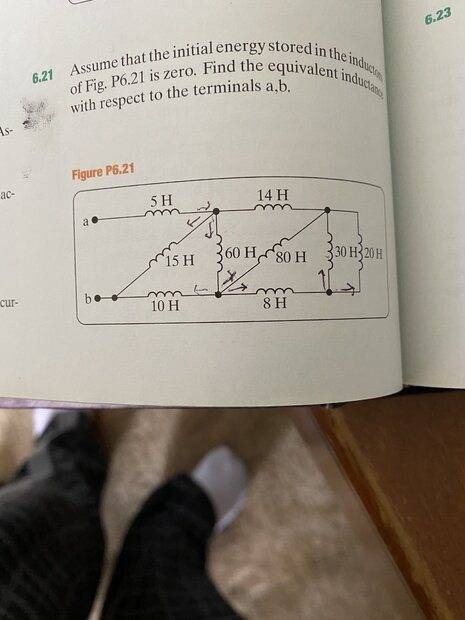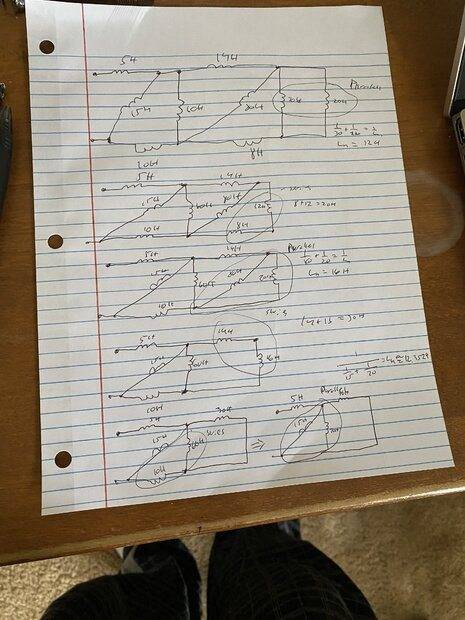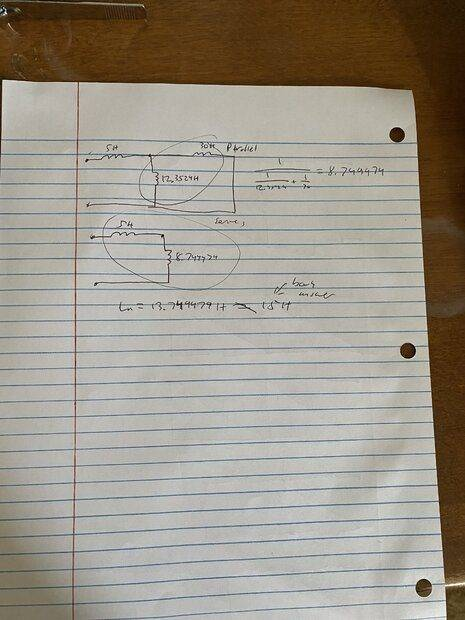# Series-Parallel Combinations of Inductors

• Engineering
• johnsmith7565

#### johnsmith7565

Homework Statement
Assume that the initial energy stored in the inductors of Fig. P6.21 is zero. Find the equivalent inductance with respect to the terminals a, b.
Relevant Equations
L(series)= L1 + L2 + ... + Ln
1/(L(parallel)) = 1/L1 + 1/L2 + ... + 1/LnI am not sure where I made the mistake. If someone could point that out that would be much appreciated!

That next-to-last step on the first page looks wrong. You are combining two resisters as though they are in series and forgetting the one in parallel on the far top-right. Instead, that step should be to combine the far top-right with the parallel one second from the right.

•DaveE
FactChecker said:
That next-to-last step on the first page looks wrong. You are combining two resisters as though they are in series and forgetting the one in parallel on the far top-right. Instead, that step should be to combine the far top-right with the parallel one second from the right.
Thanks a million! I got the correct answer.

•DaveE and FactChecker
20 // 30 = 12
+ 8 = 20
// 80 = 16
+14 = 30
// 60 = 20
+10 = 30
// 15 = 10
+ 5 = 15 H